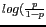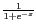DATA MINING
Desktop Survival Guide
by Graham Williams## Logistic Regression

Linear regression is a successful framework for building models. However, not all data fits the assumptions underlying linear regression.

Logistic Regression is appropriate when the target variable is binary. It is used to build a linear model involving the input variables to predict a transformation of the target variable, in particular, the logit function, which is the natural logarithm of what is called the odds'' ().

The inverse of the logit function is the logistic function,, to return the results of the linear model back to the 0-1 range.

We can see the effect of the logistic function in the following plot. Essentially, it maps numbers from a range from minus infinity to plus infinity, to the range 0 to 1.

http://rattle.togaware.com/code/rplot-logistic.R

Note: the way it goes is if it is numeric target and logistic regression it expects 0/1. If it is a categoric target, with two values, then it will convert to 0/1 internally.

Because trees are not such a numerically oriented model builder they worry less abuot value ranges.

Subsections